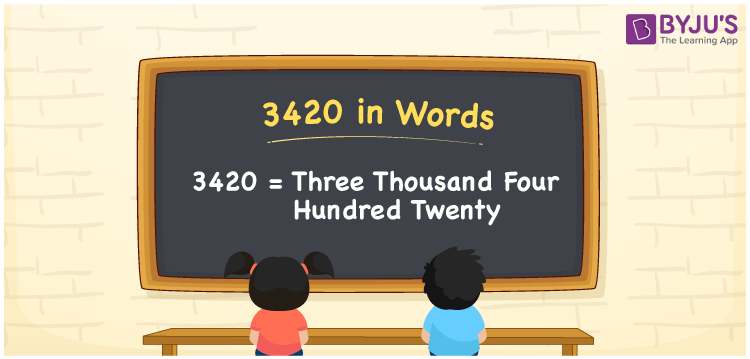# 3420 in Words

We can write the number 3420 in words as Three thousand four hundred twenty. If you have written a story of 3420 words, then you can say, “I wrote a story of Three thousand four hundred twenty words”. Thus, we can use the word form of 3420 to express or spell that number. However, we can derive the word form of the number 3420 using a place value chart. Let’s learn how to derive the number 3420 in words here in this article.

 3420 in words Three thousand four hundred twenty Three thousand four hundred twenty in Numbers 3420

## 3420 in English Words

We generally write numbers in words using the English alphabet. So, we can write 3420 in English words as “Three thousand four hundred twenty”.## How to Write 3420 in Words?

As we know, 3420 is a four-digit number, so we must make a place value chart with four columns, as shown below.

 Thousands Hundreds Tens Ones 3 4 2 0

Here, ones = 0, tens = 2, hundreds = 4, thousands = 3

Let us expand these digits according to their place values.

3 × Thousand + 4 × Hundred + 2 × Ten + 0 × One

= 3 × 1000 + 4 × 100 + 2 × 10 + 0 × 1

= 3000 + 400 + 20

= Three thousand + Four hundred + Twenty

= Three thousand four hundred twenty

Therefore, 3420 in words = Three thousand four hundred twenty.

As we know, 3420 is a natural number that precedes 3421 and succeeds 3419.

3420 in words – Three thousand four hundred twenty

Is 3420 an odd number? – No

Is 3420 an even number? – Yes

Is 3420 a perfect square number? – No

Is 3420 a perfect cube number? – No

Is 3420 a prime number? – No

Is 3420 a composite number? – Yes

## Frequently Asked Questions on 3420 in Words

Q1

### How do you write 3420 in words?

We can write the number 3420 in words as Three thousand four hundred twenty.
Q2

### How to write an amount Rs. 3420 in words?

We can express an amount Rs. 3420 in words as “Three thousand four hundred twenty rupees”.
Q3

### Find the value of Three thousand four hundred twenty minus One thousand and fifty.

Three thousand four hundred twenty minus One thousand and fifty = 3420 – 1050 = 2370